QisBool Concepts

 Q1. What an instance of QisBool ? 1.     An instance of QisBool is a single QisBool object. 2.     A QisBool object is created using QisBool_Create and destroyed using QisBool_Destroy. 3.     A QisBool object is identified by a unique handle (void*) returned when QisBool_Create executes successfully. 4.     Almost all QisBool functions require this handle to be passed as a parameter so that they know which instance of QisBool to operate upon. 5.     Each instance of QisBool object requires 1 QIS BOOLEAN license. 6.     Multiple instances of QisBool objects can co-exist in parallel. 7.     If an instance of QisBool is shared by two or more threads, the QisBool functions operating on that instance must be protected by mutual exclusion (locks/mutexes)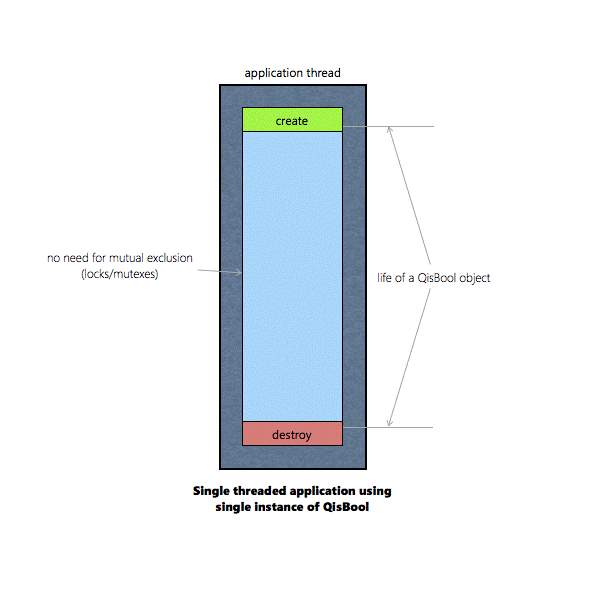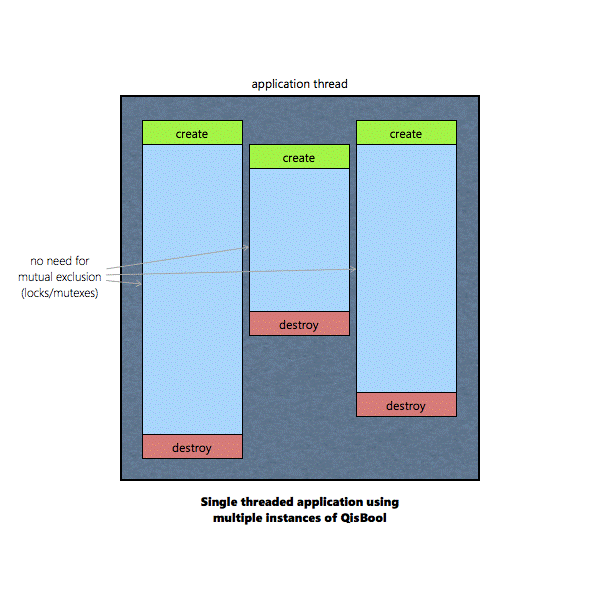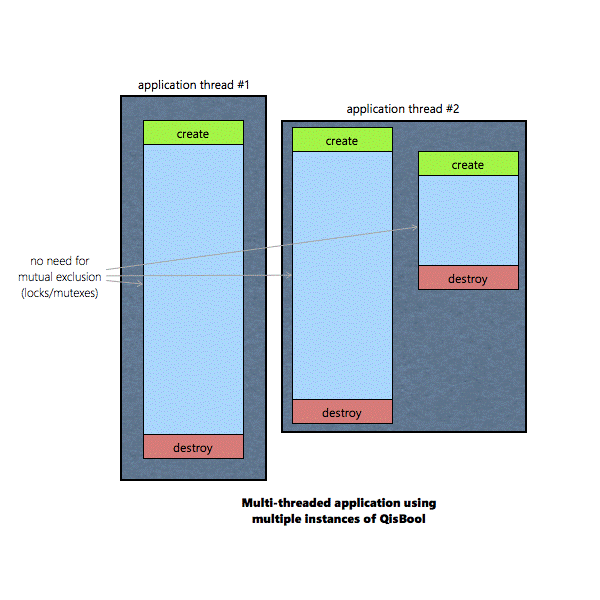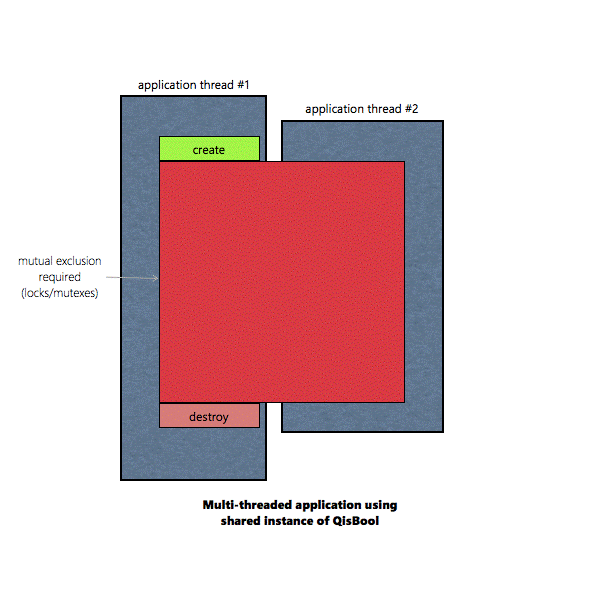QisBool Units Q1. What are the units of the geometric data used and generated by QisBool functions ? 1.     QisBool functions do not conform to any units. 2.     All geometric data used and generated by QisBool functions are represented by integers. 3.     It is the responsibility of the client code to convert the input data to from their respective units to integers based on certain acceptable 'resolution' (grid) and the re-convert the output back into the client's units using the same resolution. 4.     For example, to convert the width of a path (0.5um) to integers with a resolution of 0.001 (1000 grids per um or nm resolution), the conversion would be applied as w = 0.5/0.001 = 500. The final width passed to QisBool function would be 500. To convert a width (6578) generated by a QisBool function back to the client's units with a resolution of 0.001, w=6578*0.001 = 6.578um 5.     Similarly, to convert x,y co-ordinates of a point (123.45um, 456.789um) to integers with a resolution of 0.001, the conversion would be applied as x = 123.45/0.001 = 123450 and y = 456.789/0.001 = 456789. The final co-ordinates passed to QisBool function would be (123450, 456789). To convert an x,y co-ordinate (-890123, 7891) generated by a QisBool function back to the client's units with a resolution of 0.001, x = -890123*0.001 = -890.123 and y = 7891*0.001 = 7.891 i.e (-890.123um, 7.891um). 6.     Note: Certain QisBool functions accept geometric parameters (such as sizing amount) as doubles. This should not be confused with the units of the parameter. The geometric value must be unit less (integer) even though it is passed as a double. If a non-integral value is passed, it will be rounded off to the nearest integer either before or after the computation.

 Q1. How does QisBool represent polygons ? 1.     QisBool represents polygons using TWO pieces of information: 1. Number of Vertices (int) (NV) 2. List of x,y co-ordinates (int*) (XY) 2.     The number of vertices represents the number of points in the polygon. If the polygon is open (such as a path), the last and the first point are distinct. If the polygon is closed (such as a boundary), the last and the first point have the same x,y values. Therefore, a rectangle has 5 vertices while a single segment path as 2 vertices. 3.     The list of x,y co-ordinates is an integer array of (NV * 2) integers or NV x,y pairs. The 1st element of this array (index 0) represents the x co-ordinate of the first vertex, the 2nd element of the array (index 1) represents the y co-ordinate of the first point. Index 1 and 2 represent the second vertex and so on. 4.     The order in which the vertices appear in the list of x,y co-ordinates determines whether it is 'clock-wise' or 'counter-clockwise'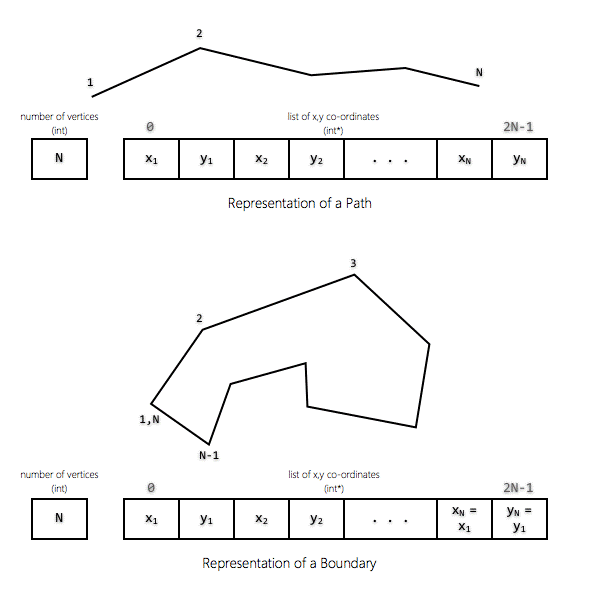1.     To represent a SET of polygons, we need THREE pieces of information: 1. Number of polygons in the set (int) (N) 2. A list of 'number of vertices' for each polygon in the set (int*) (NV) 3. A list of 'list of x,y co-ordinates' for each polygon in the set (int**) (XY) 2.     NV is an array of N integers and XY is an array of N integer arrays. If I is an index of a specific polygon in this set, then NV[I] = number of vertices in that polygon and XY[I] = list of x,y co-ordinates of that polygon. Note that number of integers in XY[I] = (NV[I] * 2) in accordance with Q1 /Pt. 3 above.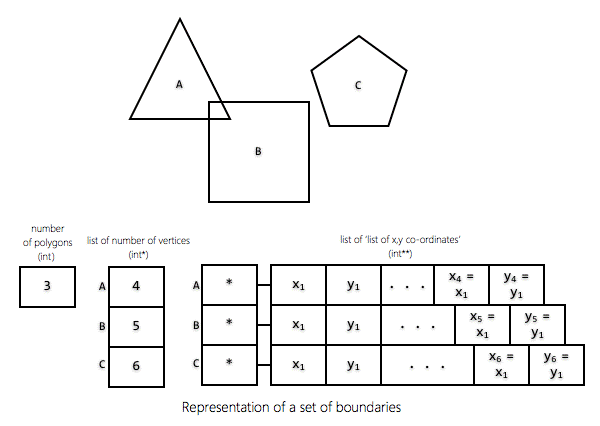Q1. What is a Leonov polygon ? 1.     A Leonov polygon is a polygon with holes. 2.     It consists of an 'outer boundary' and one or more 'holes' that lie completely inside (and do not intersect) the outer boundary. Q2. How is a Leonov polygon represented in QisBool ? 1.     A Leonov polygon is represented in QisBool as a set of polygons. 2.     The first polygon in this set represents the outer boundary or the enclosing polygon while the remaining polygons represent the holes. 3.     To differentiate the outer boundary from the holes and to differentiate a leonov polygon from a regular polygon set, the number of vertices NV for the outer boundary is negative (-1 * number of vertices). 4.     Therefore, a Leonov polygon can be represented by THREE pieces of information just like a regular polygon set: 1. Number of polygons (number of holes + outer polygon) (int) (N) 2. List of 'number of vertices' for each of those polygons (int*) (NV) 3. List of 'list of x,y co-ordinates' for each of those polygons (int**) (XY) 5.     NV and XY represent the outer boundary while NV[1..N-1] and XY[1..N-1] represent the holes. 6.     NV is negative. 7.     The only difference between the representations of Leonov polygon and a set of regular polygons is that the latter never contains a polygon with negative number of vertices. 8.     The orientation of the outer boundary is counter-clockwise while the orientation of the holes is clock-wise.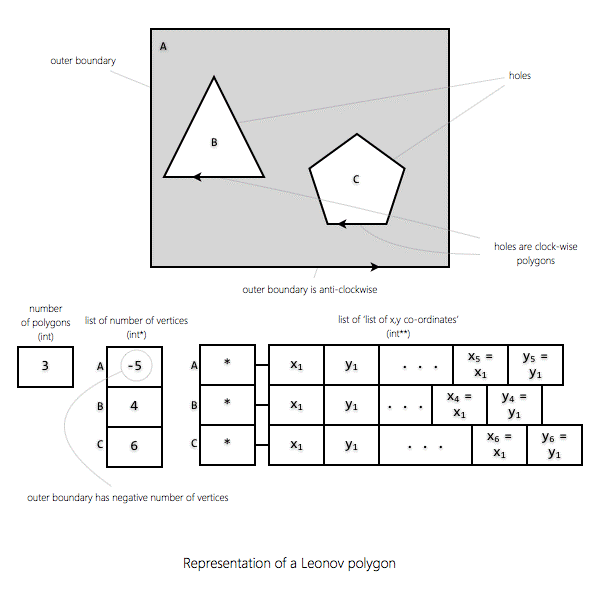Q1. What are cutlines ? 1.     Cutlines is one of the techniques used to convert a Leonov polygon into a regular polygon. 2.     Cutlines are edges that join the boundary of a hole to the outer boundary. 3.     A polygon with cutlines is a single re-entrant polygon.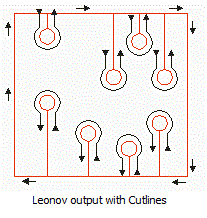Q1. What are butting polygons. 1.     Butting polygons are polygons which have one or more but not all edges that coincide. 2.     Butting is one of the techniques used to convert a leonov polygon into regular polygons without using cutlines.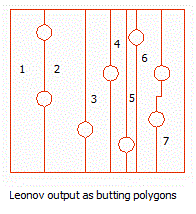Q1. What are re-entrant polygons ?. 1.     Re-entrant polygons are polygons in which at least two edges coincide. 2.     A polygon generated by using cutlines on a leonov polygon is re-entrant.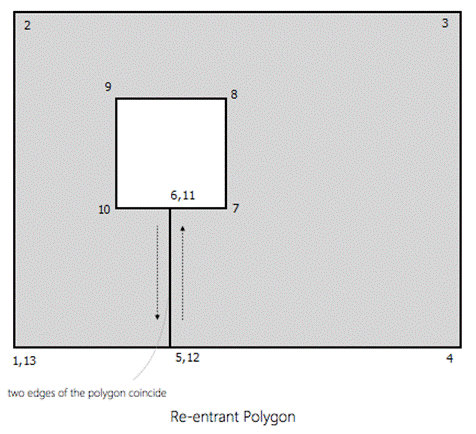Q1. What are self-touching polygons ?. 1.     Self touching polygons are polygons where either two vertices (except the first & last) coincide or a vertex lies on an edge not originating/ending on that vertex.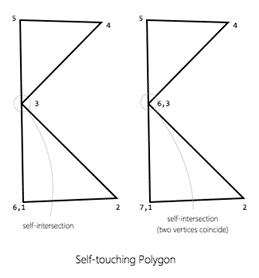Q1. What kinds of polygons should be avoided being used as input to QisBool functions ?. 1.     An open boundary (where the first vertex is not the same as the last) unless it is a path. 2.     A polygon with zero area. (a point) 3.     A one-dimensional polygon (0 width/height) unless it is a path (a line) 4.     A leonov polygon where the hole crosses the outer boundary. 5.     Self-touching polygons 6.     Re-entrant polygons 7.     Self-intersecting polygons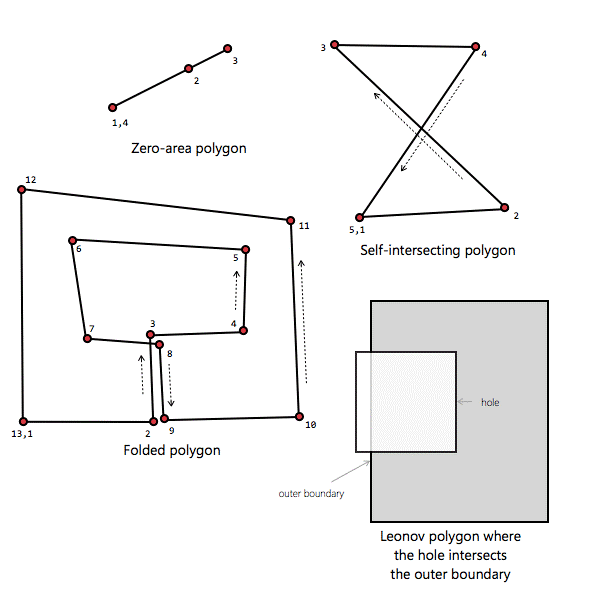Q1. Which polygon is convex in X ? 1.     A polygon is convex in X if there is no line parallel to X that intersects the polygon edges at more than TWO points. Q2. Which polygon is convex in Y ? 1.     A polygon is convex in X if there is no line parallel to Y that intersects the polygon edges at more than TWO points. Q3. Which polygon is convex in X and Y (Fully Convex) ? 1.     A polygon is fully convex if there is no line that intersects the polygon edges at more than TWO points.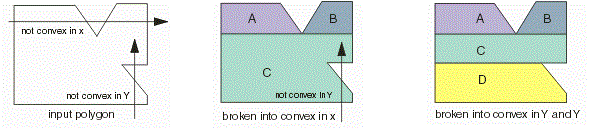Sizing Techniques Q1. What is standard sizing ? 1.     Standard sizing is a technique that applies sizing to a polygon by generating new edges that are at constant distance (sizing amount) from  the original edges. 2.     This technique is a very basic technique that does not take into account special conditions such as acute angles and may not work very well for non-fully-convex data. 3.     It may generate polygons which are considered illegal by QisBool. 4.     It is not guaranteed that all points on the new resized polygon are at same distance from the corresponding points on the original polygon. 5.     This technique must only be used for simple manhattan data.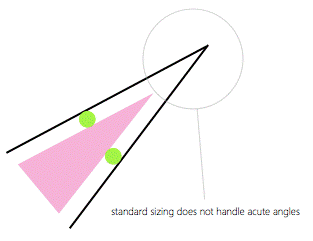Q2. What is isotropic sizing ? 1.     Isotropic sizing is a technique that applies a uniform sizing (along X and Y) to a polygon such that every point on the new resized polygon is at a uniform distance from the corresponding point on the original polygon. 2.     This is a more complex but safe-to-use form of sizing which takes care of acute angles. 3.     This technique may result in formation of arcs which can be broken up into either 1, 3 or 5 points.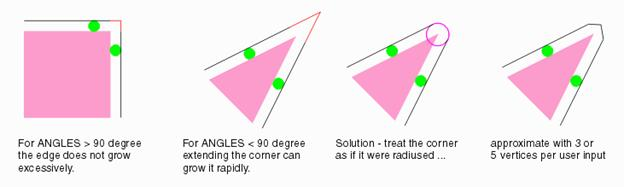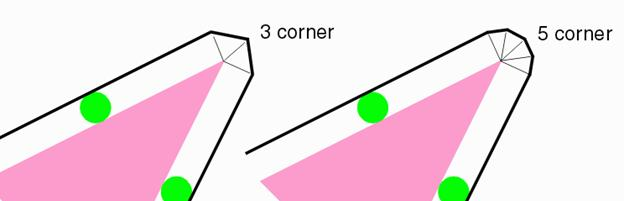Q3. What is non-isotropic sizing ? 1.     Non isotropic sizing is similar to isotropic sizing except that the amount of sizing in X and Y may not be the same.

 © 2012 Artwork Conversion Software Inc. 417 Ingalls St. Santa Cruz CA 95060 [T] +1 831-426-6163 [F] +1 831-426-2824 [E] info@artwork.com